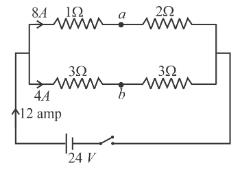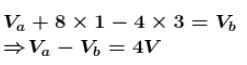Courses

# Capacitance NAT Level - 1

## 10 Questions MCQ Test Basic Physics for IIT JAM | Capacitance NAT Level - 1

Description
This mock test of Capacitance NAT Level - 1 for Physics helps you for every Physics entrance exam. This contains 10 Multiple Choice Questions for Physics Capacitance NAT Level - 1 (mcq) to study with solutions a complete question bank. The solved questions answers in this Capacitance NAT Level - 1 quiz give you a good mix of easy questions and tough questions. Physics students definitely take this Capacitance NAT Level - 1 exercise for a better result in the exam. You can find other Capacitance NAT Level - 1 extra questions, long questions & short questions for Physics on EduRev as well by searching above.
*Answer can only contain numeric values
QUESTION: 1

### In the figure given, the potential difference (in Volts) between A and B in steady state will be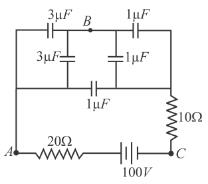Solution: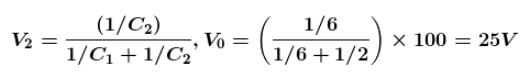*Answer can only contain numeric values
QUESTION: 2

### Three capacitors are connected as shown in figure. Then the charge (in µC) on capacitor plate C1 is :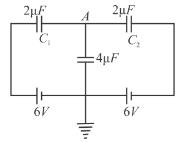Solution:

let q1 be the charge on C1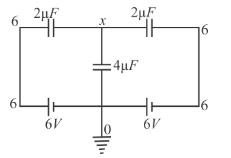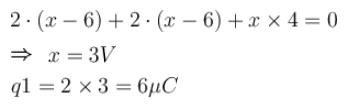*Answer can only contain numeric values
QUESTION: 3

### A capacitor of capacitance 10µF is charged to a potential of 100V. Now connecting it in parallel with an uncharged capacitor, the resultant potential difference becomes 40volt. The capacitance of this capacitor (in µF) is.

Solution: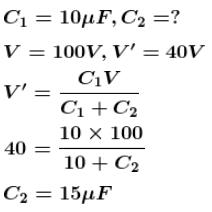*Answer can only contain numeric values
QUESTION: 4

A 4µF capacitor is charged to 400volts and then its plates are joined through a resistor of resistance 1kΩ. The heat (in Joules) produced in the resistor is

Solution:

Heat produced in the resistor =  energy stored in capacitor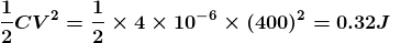*Answer can only contain numeric values
QUESTION: 5

The equivalent capacitance (in µF) in the circuit shown in figure will be.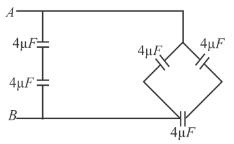Solution: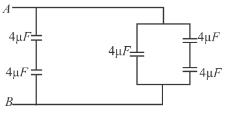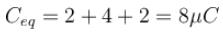*Answer can only contain numeric values
QUESTION: 6

Two capacitors of 2µF and 3µF are connected in series. The potential at point A is 1000Volt and the outer plate of 3µF capacitor is earthed. The potential at point B is (in Volts).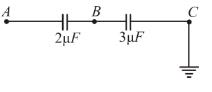Solution: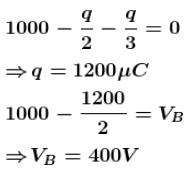*Answer can only contain numeric values
QUESTION: 7

The equivalent capacitance (in µF) of the circuit shown, between points A and B will be.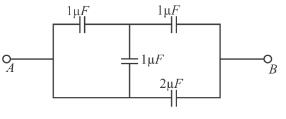Solution: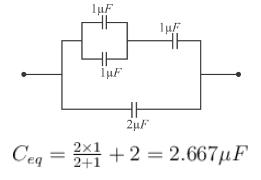*Answer can only contain numeric values
QUESTION: 8

What percentage of the energy drawn from the charging battery is stored in a capacitor.

Solution:

Energy drawn from the charge battery = QE
Energy stored in capacitor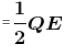So % of energy stored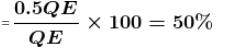*Answer can only contain numeric values
QUESTION: 9

In the circuit shown in the figure, each capacitor has a capacity of 3µF. The equivalent capacity (in µF) between A and B is.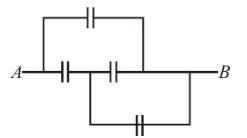Solution: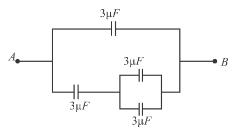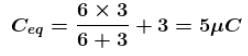*Answer can only contain numeric values
QUESTION: 10

For the circuit shown in the diagram find the value of Va – Vb  (in Volts) after long time closing the switch S1.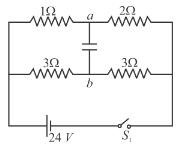Solution: